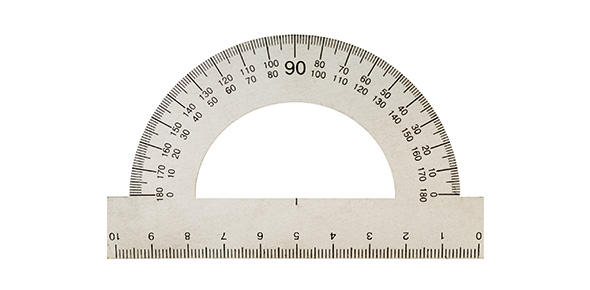# A Trivia Quiz About Inches, Feet, Pounds, And Miles

11 Questions | Attempts: 157
ShareSettingsThe United States has a distinct measuring system in comparison to other countries in the world. The system of measuring distance is based on measurements of the human body like an inch is based on the thumb, foot based on the foot etc. This quiz has been created for students to test their knowledge about inches, foot, pound and miles. Select the correct unit of measure based on the situation. So, let's try out the quiz. All the best!

• 1.
The distance between the high school and the mall would be measured with what unit?
• A.

Inches

• B.

Feet

• C.

Pounds

• D.

Miles

• 2.
The weight of yourself would be measured with what unit?
• A.

Inches

• B.

Feet

• C.

Pounds

• D.

Miles

• 3.
The length of the classroom from the door to the opposite wall would be measured with what unit?
• A.

Inches

• B.

Feet

• C.

Pounds

• D.

Miles

• 4.
The length of your math textbook would be measured using what unit of measure?
• A.

Inches

• B.

Feet

• C.

Pounds

• D.

Miles

• 5.
The length of the hallway between the cafeteria and the classroom would be best measured by what unit of measure?
• A.

Inches

• B.

Feet

• C.

Pounds

• D.

Miles

• 6.
Which choice best represents the height at which a helicopter flies?
• A.

1000 miles

• B.

1000 inches

• C.

1000 feet

• D.

1000 yards

• 7.
Which choice best represents the height of an electric pole?
• A.

18 feet

• B.

18 inches

• C.

18 miles

• D.

18 yards

• 8.
Which choice best represents the length of a book?
• A.

10 miles

• B.

10 inches

• C.

10 yards

• D.

10 feet

• 9.
Which choice best represents the length of a toothpick?
• A.

2 feet

• B.

2 miles

• C.

2 yards

• D.

2 inches

• 10.
Which choice best represents the height of a hill?
• A.

1000 feet

• B.

1000 yards

• C.

1000 inches

• D.

1000 miles

• 11.
Which choice best represents the height of a can of energy drink?
• A.

4 miles

• B.

4 feet

• C.

4 inches

• D.

4 yards

## Related TopicsBack to top
×

Wait!
Here's an interesting quiz for you.